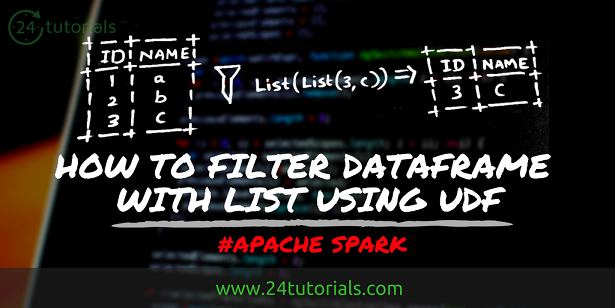Spark

# How to filter DataFrame based on keys in Scala List using Spark UDF [Code Snippets]There are some situations where you are required to Filter the Spark DataFrame based on the keys which are already available in Scala collection.

Let’s see how we can achieve this in Spark. You need to use spark UDF for this –

Step -1: Create a DataFrame using parallelize method by taking sample data.

```scala> val df = sc.parallelize(Seq((2,"a"),(3,"b"),(5,"c"))).toDF("id","name")
df: org.apache.spark.sql.DataFrame = [id: int, name: string]```

Step -2: Create a UDF which concatenates columns inside dataframe. Below UDF accepts a collection of columns and returns concatenated column separated by the given delimiter.

```scala> val concatKey = udf( (xs: Seq[Any], sep:String) => xs.filter(_ != null).mkString(sep))
concatKey: org.apache.spark.sql.UserDefinedFunction = UserDefinedFunction(,StringType,List())```

Step -3: Add a new column and apply UDF created above which yields concatenated column. Here array is a utility available in Spark framework which holds a collection of spark columns.

```scala> val df_with_concatfield = df.withCoumn("concat_id_name", concatKey(array("id","name"),lit("-")))
df_with_concatfield: org.apache.spark.sql.DataFrame = [id: int, name: string, concat_id_name: string]```
```scala> df_with_concatfield.show
+---+----+--------------+
| id|name|concat_id_name|
+---+----+--------------+
|  2|   a|           2-a|
|  3|   b|           3-b|
|  5|   c|           5-c|
+---+----+--------------+```

Step -4: Consider below are the keys available in the List which used to filter the dataframe. Concat keys inside list using map function to get combination keys.

```scala> val keyList = List(List(1,"a"),List(3,"b"))
keyList: List[List[Any]] = List(List(1, a), List(3, b))```
```scala> val concat_keyList = keyList.map(_.mkString("-"))
concat_keyList: List[String] = List(1-a, 3-b))```

Step -5: Use filter-isin conditions utilities on the above created dataframe and filter based on the list as shown below –

```scala> val filtered_df = df_with_concatfield.filter(df_with_concatfield("concat_id_name".isin(concat_keyList:_*)))
filtered_df: org.apache.spark.sql.DataFrame = [id: int, name:string, concat_id_name: string]```
```scala> filtered_df.show
+---+----+--------------+
| id|name|concat_id_name|
+---+----+--------------+
|  3|   b|           3-b|
+---+----+--------------+```